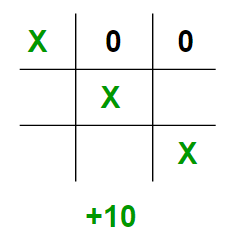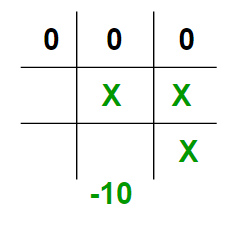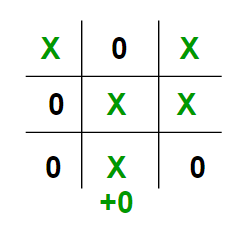Open In App

# Introduction to Evaluation Function of Minimax Algorithm in Game Theory

Prerequisite: Minimax Algorithm in Game Theory
As seen in the above article, each leaf node had a value associated with it. We had stored this value in an array. But in the real world when we are creating a program to play Tic-Tac-Toe, Chess, Backgammon, etc. we need to implement a function that calculates the value of the board depending on the placement of pieces on the board. This function is often known as Evaluation Function. It is sometimes also called a Heuristic Function.
The evaluation function is unique for every type of game. In this post, the evaluation function for the game Tic-Tac-Toe is discussed. The basic idea behind the evaluation function is to give a high value for a board if the maximizer turn or a low value for the board if the minimizer turn.
For this scenario let us consider X as the maximizer and O as the minimizer.
Let us build our evaluation function :

• If X wins on the board we give it a positive value of +10.• If O wins on the board we give it a negative value of -10.• If no one has won or the game results in a draw then we give a value of +0.We could have chosen any positive/negative. For the sake of simplicity, we chose 10 and shall use lowercase ‘x’ and lowercase ‘o’ to represent the players and an underscore ‘_’ to represent a blank space on the board.
If we represent our board as a 3×3 2D character matrix, like char board; then we have to check each row, each column, and the diagonals to check if either of the players has gotten 3 in a row.

## C++

 `// C++ program to compute evaluation function for``// Tic Tac Toe Game.``#include``#include``using` `namespace` `std;` `// Returns a value based on who is winning``// b is the Tic-Tac-Toe board``int` `evaluate(``char` `b)``{``    ``// Checking for Rows for X or O victory.``    ``for` `(``int` `row = 0; row<3; row++)``    ``{``        ``if` `(b[row]==b[row] && b[row]==b[row])``        ``{``            ``if` `(b[row]==``'x'``)``               ``return` `+10;``            ``else` `if` `(b[row]==``'o'``)``               ``return` `-10;``        ``}``    ``}` `    ``// Checking for Columns for X or O victory.``    ``for` `(``int` `col = 0; col<3; col++)``    ``{``        ``if` `(b[col]==b[col] && b[col]==b[col])``        ``{``            ``if` `(b[col]==``'x'``)``                ``return` `+10;``            ``else` `if` `(b[col]==``'o'``)``                ``return` `-10;``        ``}``    ``}` `    ``// Checking for Diagonals for X or O victory.``    ``if` `(b==b && b==b)``    ``{``        ``if` `(b==``'x'``)``            ``return` `+10;``        ``else` `if` `(b==``'o'``)``            ``return` `-10;``    ``}``    ``if` `(b==b && b==b)``    ``{``        ``if` `(b==``'x'``)``            ``return` `+10;``        ``else` `if` `(b==``'o'``)``            ``return` `-10;``    ``}` `    ``// Else if none of them have won then return 0``    ``return` `0;``}` `// Driver code``int` `main()``{``    ``char` `board =``    ``{``        ``{ ``'x'``, ``'_'``, ``'o'``},``        ``{ ``'_'``, ``'x'``, ``'o'``},``        ``{ ``'_'``, ``'_'``, ``'x'``}``    ``};` `    ``int` `value = evaluate(board);``    ``printf``(``"The value of this board is %d\n"``, value);``    ``return` `0;``}`

## Java

 `// Java program to compute evaluation function for``// Tic Tac Toe Game.` `class` `GFG``{` `// Returns a value based on who is winning``// b is the Tic-Tac-Toe board``static` `int` `evaluate(``char` `b[][])``{``    ``// Checking for Rows for X or O victory.``    ``for` `(``int` `row = ``0``; row < ``3``; row++)``    ``{``        ``if` `(b[row][``0``] == b[row][``1``] && b[row][``1``] == b[row][``2``])``        ``{``            ``if` `(b[row][``0``] == ``'x'``)``            ``return` `+``10``;``            ``else` `if` `(b[row][``0``] == ``'o'``)``            ``return` `-``10``;``        ``}``    ``}` `    ``// Checking for Columns for X or O victory.``    ``for` `(``int` `col = ``0``; col < ``3``; col++)``    ``{``        ``if` `(b[``0``][col] == b[``1``][col] && b[``1``][col] == b[``2``][col])``        ``{``            ``if` `(b[``0``][col] == ``'x'``)``                ``return` `+``10``;``            ``else` `if` `(b[``0``][col] == ``'o'``)``                ``return` `-``10``;``        ``}``    ``}` `    ``// Checking for Diagonals for X or O victory.``    ``if` `(b[``0``][``0``] == b[``1``][``1``] && b[``1``][``1``] == b[``2``][``2``])``    ``{``        ``if` `(b[``0``][``0``] == ``'x'``)``            ``return` `+``10``;``        ``else` `if` `(b[``0``][``0``] == ``'o'``)``            ``return` `-``10``;``    ``}``    ``if` `(b[``0``][``2``] == b[``1``][``1``] && b[``1``][``1``] == b[``2``][``0``])``    ``{``        ``if` `(b[``0``][``2``] == ``'x'``)``            ``return` `+``10``;``        ``else` `if` `(b[``0``][``2``] == ``'o'``)``            ``return` `-``10``;``    ``}` `    ``// Else if none of them have won then return 0``    ``return` `0``;``}` `// Driver code``public` `static` `void` `main(String[] args)``{``    ``char` `board[][] =``    ``{``        ``{ ``'x'``, ``'_'``, ``'o'``},``        ``{ ``'_'``, ``'x'``, ``'o'``},``        ``{ ``'_'``, ``'_'``, ``'x'``}``    ``};` `    ``int` `value = evaluate(board);``    ``System.out.printf(``"The value of this board is %d\n"``, value);``}``}` `// This code is contributed by PrinciRaj1992`

## Python3

 `# Python3 program to compute evaluation``# function for Tic Tac Toe Game.` `# Returns a value based on who is winning``# b is the Tic-Tac-Toe board``def` `evaluate(b):`` ` `    ``# Checking for Rows for X or O victory.``    ``for` `row ``in` `range``(``0``, ``3``):``     ` `        ``if` `b[row][``0``] ``=``=` `b[row][``1``] ``and` `b[row][``1``] ``=``=` `b[row][``2``]:``         ` `            ``if` `b[row][``0``] ``=``=` `'x'``:``                ``return` `10``            ``else` `if` `b[row][``0``] ``=``=` `'o'``:``                ``return` `-``10` `    ``# Checking for Columns for X or O victory.``    ``for` `col ``in` `range``(``0``, ``3``):``     ` `        ``if` `b[``0``][col] ``=``=` `b[``1``][col] ``and` `b[``1``][col] ``=``=` `b[``2``][col]:``         ` `            ``if` `b[``0``][col]``=``=``'x'``:``                ``return` `10``            ``else` `if` `b[``0``][col] ``=``=` `'o'``:``                ``return` `-``10` `    ``# Checking for Diagonals for X or O victory.``    ``if` `b[``0``][``0``] ``=``=` `b[``1``][``1``] ``and` `b[``1``][``1``] ``=``=` `b[``2``][``2``]:``     ` `        ``if` `b[``0``][``0``] ``=``=` `'x'``:``            ``return` `10``        ``else` `if` `b[``0``][``0``] ``=``=` `'o'``:``            ``return` `-``10``     ` `    ``if` `b[``0``][``2``] ``=``=` `b[``1``][``1``] ``and` `b[``1``][``1``] ``=``=` `b[``2``][``0``]:``     ` `        ``if` `b[``0``][``2``] ``=``=` `'x'``:``            ``return` `10``        ``else` `if` `b[``0``][``2``] ``=``=` `'o'``:``            ``return` `-``10``     ` `    ``# Else if none of them have won then return 0``    ``return` `0`` ` `# Driver code``if` `__name__ ``=``=` `"__main__"``:`` ` `    ``board ``=` `[[``'x'``, ``'_'``, ``'o'``],``             ``[``'_'``, ``'x'``, ``'o'``],``             ``[``'_'``, ``'_'``, ``'x'``]]``     ` `    ``value ``=` `evaluate(board)``    ``print``(``"The value of this board is"``, value)` `# This code is contributed by Rituraj Jain`

## C#

 `// C# program to compute evaluation function for``// Tic Tac Toe Game.``using` `System;` `class` `GFG``{` `// Returns a value based on who is winning``// b[3,3] is the Tic-Tac-Toe board``static` `int` `evaluate(``char` `[,]b)``{``    ``// Checking for Rows for X or O victory.``    ``for` `(``int` `row = 0; row < 3; row++)``    ``{``        ``if` `(b[row, 0] == b[row, 1] && b[row, 1] == b[row, 2])``        ``{``            ``if` `(b[row, 0] == ``'x'``)``            ``return` `+10;``            ``else` `if` `(b[row, 0] == ``'o'``)``            ``return` `-10;``        ``}``    ``}` `    ``// Checking for Columns for X or O victory.``    ``for` `(``int` `col = 0; col < 3; col++)``    ``{``        ``if` `(b[0, col] == b[1, col] && b[1, col] == b[2, col])``        ``{``            ``if` `(b[0, col] == ``'x'``)``                ``return` `+10;``            ``else` `if` `(b[0, col] == ``'o'``)``                ``return` `-10;``        ``}``    ``}` `    ``// Checking for Diagonals for X or O victory.``    ``if` `(b[0, 0] == b[1, 1] && b[1, 1] == b[2, 2])``    ``{``        ``if` `(b[0, 0] == ``'x'``)``            ``return` `+10;``        ``else` `if` `(b[0, 0] == ``'o'``)``            ``return` `-10;``    ``}``    ``if` `(b[0, 2] == b[1, 1] && b[1, 1] == b[2, 0])``    ``{``        ``if` `(b[0, 2] == ``'x'``)``            ``return` `+10;``        ``else` `if` `(b[0, 2] == ``'o'``)``            ``return` `-10;``    ``}` `    ``// Else if none of them have won then return 0``    ``return` `0;``}` `// Driver code``public` `static` `void` `Main(String[] args)``{``    ``char` `[,]board =``    ``{``        ``{ ``'x'``, ``'_'``, ``'o'``},``        ``{ ``'_'``, ``'x'``, ``'o'``},``        ``{ ``'_'``, ``'_'``, ``'x'``}``    ``};` `    ``int` `value = evaluate(board);``    ``Console.Write(``"The value of this board is {0}\n"``, value);``}``}` `// This code is contributed by Rajput-Ji`

## Javascript

 ``

Output

`The value of this board is 10`

Time Complexity: O(max(row,col))
Auxiliary Space: O(1)

The idea of this article is to understand how to write a simple evaluation function for the game Tic-Tac-Toe. In the next article we shall see how to combine this evaluation function with the minimax function. Stay Tuned.